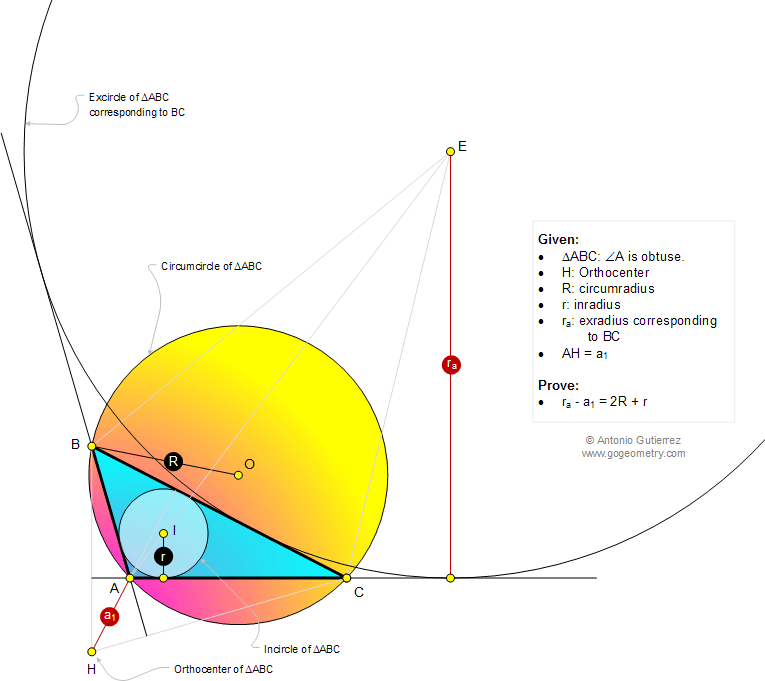# Infographic Geometry Problem 1066: Triangle, Obtuse Angle, Orthocenter, Circumradius, Inradius, Exradius, Distance, Diameter. Level: High School, SAT Prep, College, Mathematics Education

< PREVIOUS PROBLEM  |  NEXT PROBLEM >

 The figure shows a triangle ABC with obtuse angle A, the orthocenter H, the circumradius R, the inradius r, and the exradius ra corresponding to side BC. If AH =a1, prove that ra - a1 = 2R + r.Home | Search | Geometry | Problems | All Problems | Open Problems | Visual Index | 10 Problems | 1061-1070 | Triangle | Orthocenter | Altitude | Circle | Circumradius | Inradius | Exradius | Email | by Antonio Gutierrez Add or view a solution to the problem 1066 Last updated: Dec 7, 2014